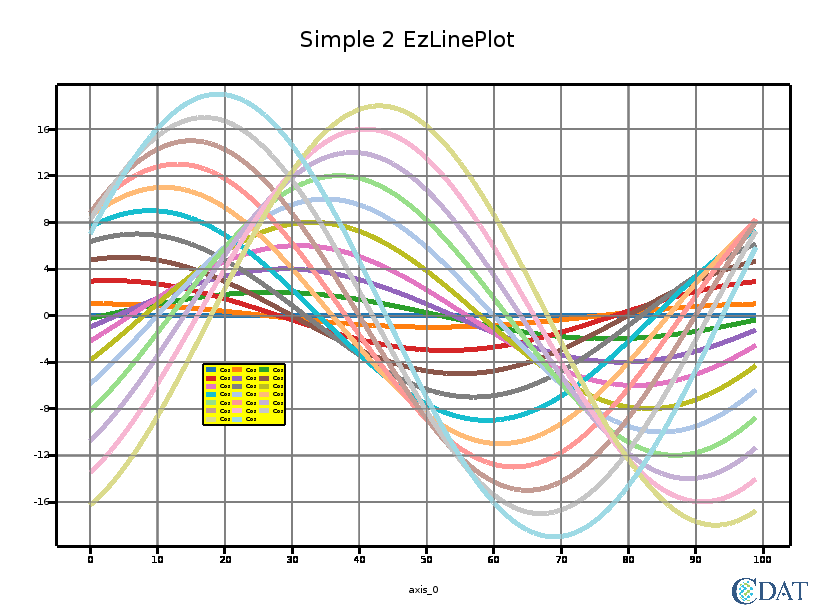# EzPlot Example¶

To download this Jupyter Notebook, right click on the link and choose "Download Linked File As..." or "Save Link as...". Remember where you saved the downloaded file, which should have an .ipynb extension. (You'll need to launch the Jupyter notebook or JupyterLab instance from the location where you store the notebook file.)

# Simple Plot¶

Back to Top

In :
import os
import vcs
import cdms2
import vcsaddons.EzPlot
import cdutil
import math
import random

# Simple Plot with EzPlot

variables  = []
line_type  = []
line_widths = []
legend_texts = []
legend_text_colors = []
marker_type = []

for v in range(0, 20):
var = []
for i in range(0, 100):
if v%2 == 0:
#var.append(random.random() * v * math.sin(2*math.pi*float(i-v)/100.0))
var.append(v * math.sin(2*math.pi*float(i-v)/100.0))
else:
#var.append(random.random() * v * math.cos(2*math.pi*float(i-v)/100.0))
var.append(v * math.cos(2*math.pi*float(i-v)/100.0))
line_type += ["solid"]
line_widths += 
if i%2 == 0:
legend_texts += ["Sin"]
else:
legend_texts += ["Cos"]
legend_text_colors += ['black']
marker_type += [None]
variables.append(var)

plotfunction = vcsaddons.EzPlot.EzLinePlot()

canvas = vcs.init()

d = plotfunction.lineplot(data=variables, canvas=canvas, title="Simple 2 EzLinePlot", titlesize=30,
#backgroundcolor=[0, 100, 0],
linetypes=line_type, linewidths=line_widths,
legendposition=[0.25, 0.35, 0.30, 0.40], legendtextcolors=legend_text_colors,
legendtexts=legend_texts,
legendbackgroundcolor="yellow",
enablegrid=True)

canvas.png("EzPlot_Simple2")

d

Out:The CDAT software was developed by LLNL. This tutorial was written by Charles Doutriaux. This work was performed under the auspices of the U.S. Department of Energy by Lawrence Livermore National Laboratory under Contract DE-AC52-07NA27344.

If you have questions about this notebook, please email our CDAT Support address, cdat-support@llnl.gov.